# P-Value In ExcelArticle byExcelMojo Team## What Is P-Value In Excel?

The P-Value in Excel is the probability value that denotes the extent, an observation or finding is statistically significant. And it ranges between 0 and 1.

Users can use the P-Value to decide whether to accept or reject a null hypothesis while performing hypothesis testing during analyses and tests such as Regression and T-Test.

For example, the below table contains the height ranges of two plant types.

Suppose we must perform a paired, two-sample T-Test to determine whether the mean values of the two given datasets have a notable difference. Assume the target cell to display the T-Test result is cell B13.

And consider the null hypothesis is that the mean values of the two datasets are the same.

Then, the solution is to find P-Value in Excel cell B13 using the T.TEST() to decide whether to accept or deny the null hypothesis.

The T.TEST() output is 0.028738184, the statistical significance P Value in Excel.

And the value is below the significance threshold of 0.05, implying the chances of the two data sets’ mean values being the same is less than 5%.

It interprets that the difference in the mean values of the two samples is considerable. And thus, the null hypothesis is false, and we need to reject it.

###### Key Takeaways
• The P-Value in Excel is a probability value ranging from 0 to 1. It shows how much a finding is statistically considerable.
• Users can use the P-Value determined during analyses and tests, such as Regression, Anova, and T-Test, to perform hypothesis testing.
• While you can perform the T-Test using the Excel inbuilt function, T.TEST(), or Data Analysis ToolPak to obtain the P-Value, the TDIST() is the P-Value Excel formula.
• If the determined P-Value is less than or equal to the default significance threshold of 0.05, the given data is significant. Otherwise, the specified data is not significant.

### P-Value Formula In Excel

The T-Test() syntax is:

where,

• array1: The first dataset.
• array2: The second dataset.
• tails: The distribution tail applied in a T-Test. A value of 1 denotes a one-tailed distribution. And a value of 2 indicates a two-tailed distribution.
• type: The T-Test type. The argument can take the values 1, 2, or 3, indicating Paired T-Test, Two-sample equal and unequal variance T-Tests, respectively.

All the arguments in the above T.TEST() syntax are mandatory.

But while applying the T.TEST() gives the probability associated with the T-Test, the formula for P-Value in Excel is TDIST().

The TDIST() syntax is:

where,

• x: A calculated numeric value of t at which we must determine the student’s t-distribution.
• deg_freedom: An integer signifying the number of degrees of freedom.
• tails: The distribution tails count require the TDIST() to return. While 1 denotes a one-tailed t-distribution, 2 indicates a two-tailed t-distribution.

All three arguments in the above TDIST() syntax are mandatory.

Further, for a non-numeric argument value, x value below 0, deg_freedom below 1, or tails not being 1 or 2, the TDIST() throws errors such as the #VALUE! and #NUM!. Thus, in such cases, we might face the condition of the P-Value in Excel not working.

### How To Calculate P-Value In Excel?

We can calculate the P-Value in Excel in the following two ways:

1. Access T.TEST() and TDIST() from the Excel ribbon.
2. Enter T.TEST() and TDIST() in the worksheet manually.

#### Method #1 – Access From The Excel Ribbon

• T.TEST()

Choose the target cell for output and select FormulasMore Functions option drop-down → Statistical option right arrow → T.TEST function, as shown below.

The Function Arguments window appears. Enter the arguments in the Array1, Array2, Tails, and the Type fields → click OK, as shown below.

• TDIST()

Choose a target cell for output and select FormulasMore Functions option drop-down → Compatibility option right arrow → TDIST function, as shown below.

The Function Arguments window appears. Enter the arguments in the X, Deg_freedom, and the Tails fields → click OK, as depicted below.

#### Method #2 – Enter In The Worksheet Manually

• T.TEST()
1. To begin with, select an empty cell for the output.
2. Next, type =T.TEST( in the cell. [Alternatively, type =T.T and double-click the T.TEST excel function from the Excel suggestions.]
3. Then, enter the arguments as cell values or references and close the brackets.
4. Press Enter to execute the formula and get the required P-Value.
• TDIST()
1. First, select an empty cell for the output.
2. Type =TDIST( in the cell. [Alternatively, type =TD and double-click the TDIST function from the Excel suggestions.]
3. Then, enter the arguments as cell values or references and close the brackets.
4. Press Enter to execute the formula and get the required P-Value.

• T.TEST()

The below table contains the target data of two sales teams.

The two given independent data sets have the same population variation. And suppose the null hypothesis is that the two data sets have the same mean value.

So, we shall perform the T-Test for a one-tailed distribution to get the P-Value in Excel T-Test and decide whether to accept or reject the null hypothesis. Assume the target cell is B13.

1. To begin with, select the target cell B13, enter the T.TEST() in the following method:

Then, start typing in the formula and provide the two array ranges, as depicted below.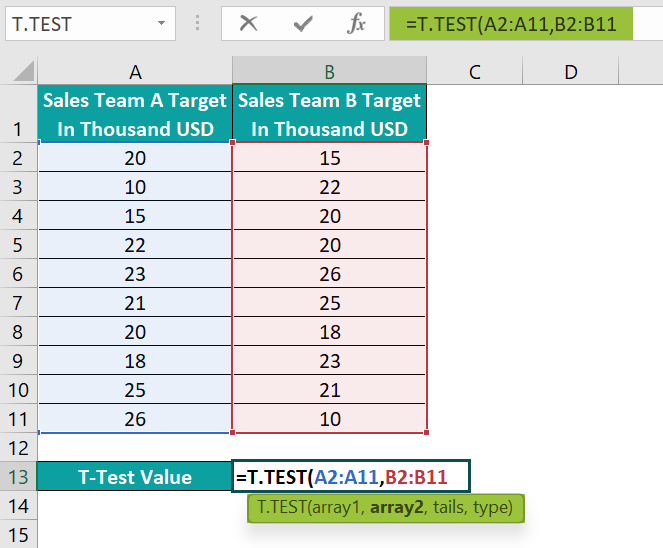2. Next, enter a comma, and Excel will show us the tails options to select. Double-click on the first option, as it is a one-tailed T-Test.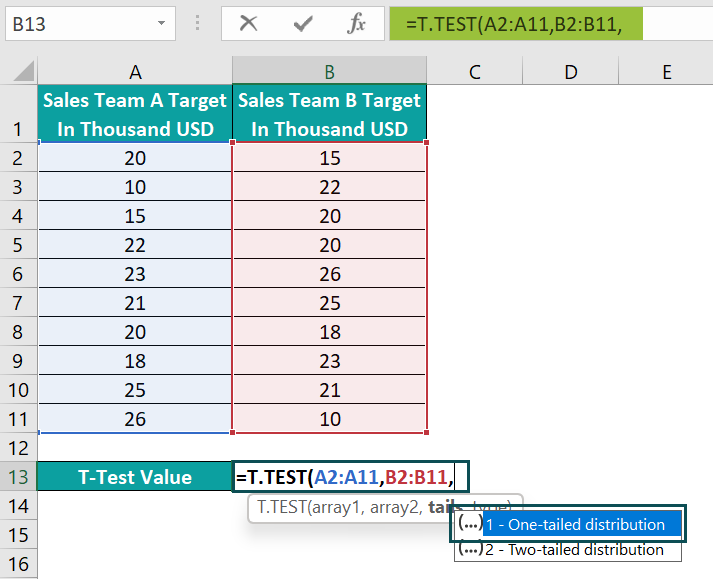And then, enter a comma, after which Excel will show the type options to select. Double-click on the second option, as the data sets have the same variance.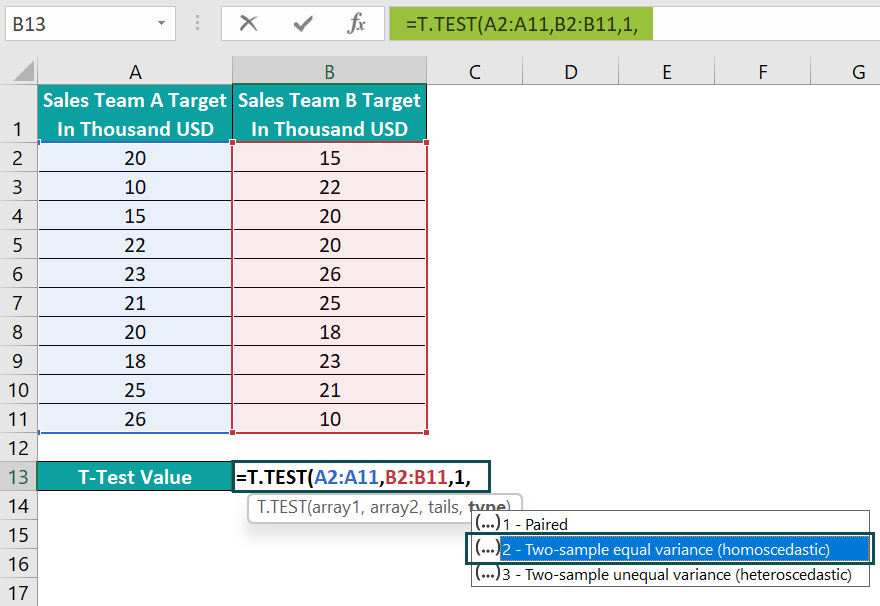3. Finally, close the parenthesis and then, press Enter to execute the formula.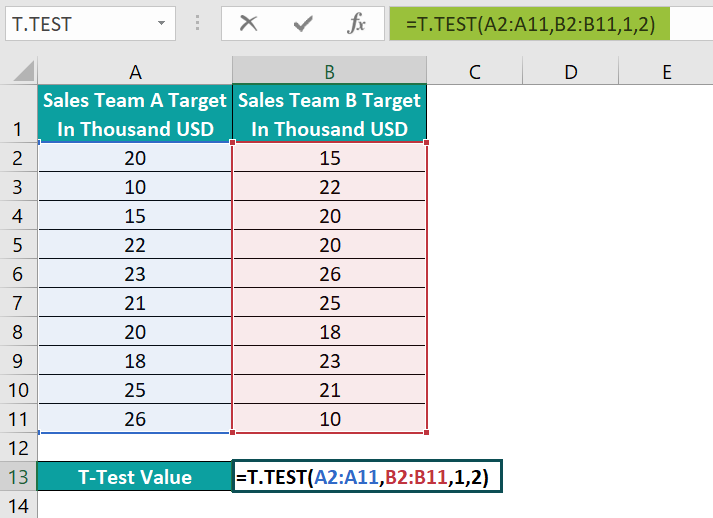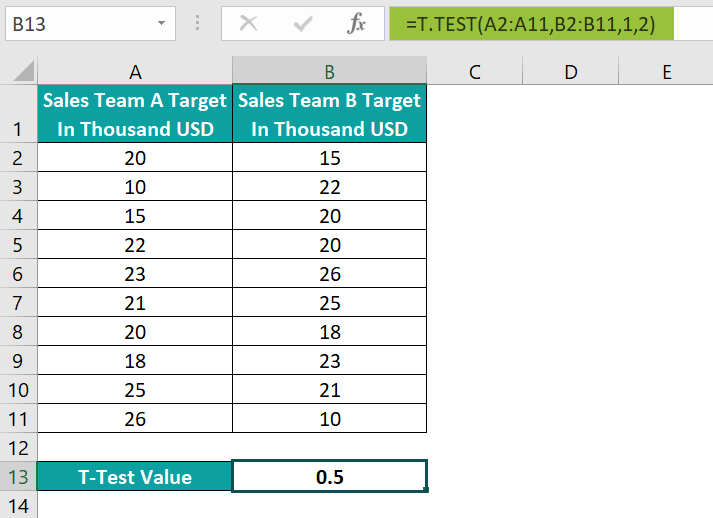On the other hand, entering the third and fourth arguments directly as the function arguments will also give the same result.

Alternatively, select the target cell B13 and go to FormulasMore FunctionsStatisticalT.TEST to apply the function in the chosen cell.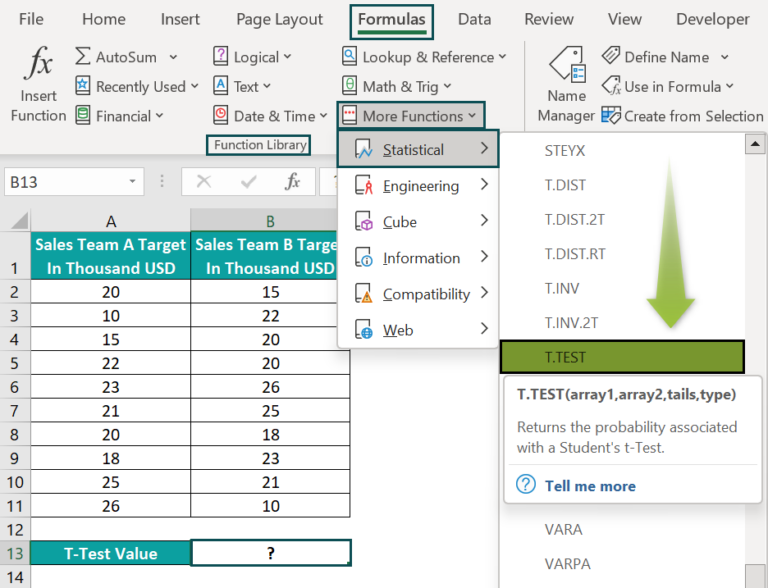The above step will open the Function Arguments window.

Next, enter the given cell ranges and references in the respective fields in the Function Arguments window.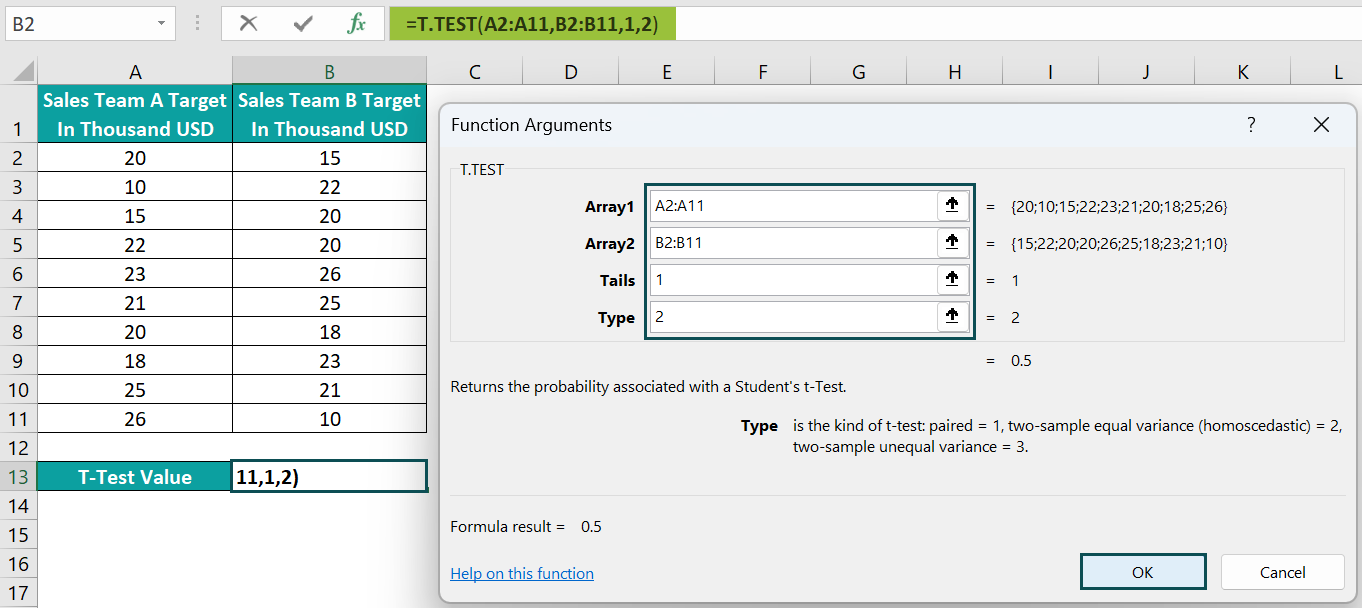Finally, click OK to close the window, and we will see the T.TEST() output in the target cell B13.

Alternatively, we can use the Data Analysis (Analysis ToolPak) in the Data tab to perform the T-Test and find P-Value in Excel.

But first, we must install the Analysis ToolPak to access the Data Analysis option in Excel.

• Step 1: Click FileOptions to open the Excel Options window.
• Step 2: Go to the Add-ins tab, click on the inactive application Analysis ToolPak, and Go.
• Step 3: The Add-ins window appears where we must check the Analysis ToolPak box.

And once we click OK, the Data Analysis feature will appear in the Data tab.

We shall now see the steps to use the Data Analysis option to get the P-Value in Excel T-Test.

• Step 1: Navigate through DataData Analysis.
• Step 2: The Data Analysis window opens, where we must select the T-Test required to determine the statistical significance P Value in Excel for datasets with equal variance. And click OK to open the required T-Test window.

Ensure to pick the appropriate T-Test to avoid the condition of P-Value in Excel not working.

• Step 3: Enter the two value ranges, including the column headers, since we select the Labels option to show the respective column headers in the output. And the Alpha or significance threshold is 0.05.

Also, we can enter the reference to the target cell E1 as the Output Range to display the output in the same sheet.

• Step 4: Click OK to view the specified T-Test result.

The T.TEST() and the T-Test in the Data Analysis ToolPak give the same P-Value (one-tail) of 0.5.

The inference is that since the obtained P-Value of 0.5 is more than the significance threshold of 0.05, we cannot reject the null hypothesis. And thus, there is inadequate proof to conclude that the mean values of the two data sets are not the same. In other words, the computed P-Value indicates a high probability that the two data sets have the same mean value.

• TDIST()

The below table shows the TDIST() arguments values required to calculate the student’s one-tailed t-distribution probability percentage in cell B8.

Assume the target cell data format is Percentage in Home à Number Format. Then, here is how we can apply the TDIST() in the target cell to get the P-Value.

• Step 1: To start with, select the target cell B8, enter the TDIST() in the following method:

First, start entering the formula and provide the first two argument values.

Next, enter a comma, and Excel will display the tails options to select. And as it is a one-tailed T-distribution, double-click on the first option.

And then close the parenthesis.

Finally, press Enter to execute the TDIST().

Further, entering the value one or cell reference in excel to the value one as the third argument in the TDIST() will give the same output.

=TDIST(C3,C4,C5)

Alternatively, select the target cell B8 and go to FormulasMore FunctionsCompatibilityTDIST to apply the function in the chosen cell.

The above step will open the Function Arguments window.

Next, enter the given cell references in the respective fields in the Function Arguments window.

Finally, click OK to close the window, and view the TDIST() output in the target cell B8.

The TDIST() returns the student’s one-tailed t-distribution probability for exact 0.9 and the given degree of freedom, 5, as 0.2046856, which is 20.47%.

### Examples

We shall see a few examples of P-Value in Excel.

#### Example #1

This example explains one-sample T-Test. We can determine the probability of the sample mean value differing considerably from a hypothesized mean.

The first table contains the monthly high-temperature data for a city in Texas.

Hypothetically, consider the average high temperature across all the months in the city is 70°F.

We shall perform the one-sample T-Test to determine whether the high-temperature values in the sample data differ significantly from the hypothesized average high temperature. And we will use the second table to perform the one-sample T-Test calculations and get the P-Value in cell F10.

The null and alternative hypotheses are:

• Null hypothesis: No significant difference between the sample high-temperature values and the average high temperature.
• Alternate hypothesis: Significant difference between the sample high-temperature values and the average high temperature.
• Step 1: First, select cell F3, enter the AVERAGE excel function to determine the sample mean (), and then, press Enter.

=AVERAGE(B3:B14)

• Step 2: Next, choose cell F4, enter the STDEV.S() to find the sample standard deviation formula, and then, press Enter.

=STDEV.S(B3:B14)

• Step 3: Select cell F5, enter the COUNT excel function to get the total data points in the sample, and press Enter.

=COUNT(B3:B14)

• Step 4: Next, we need to calculate the standard error in mean (SEM) using the below mathematical expression:

Where,

• s: Standard Deviation
• n: Total number of data points in the sample

So, select cell F6, enter the below formula, and press Enter.

=F4/SQRT(F5)

• Step 5: Select cell F7, enter the below formula to calculate the degree of freedom, and press Enter.

=F5-1

• Step 6: Select cell F8, enter the hypothesized mean value, µ, as 70, and press Enter.
• Step 7: Next, select cell F9 to enter the t-statistic value, with the mathematical formula being:

where,

• x̅ : Sample mean
• µ : Hypothesized mean (70°F in this example)
• s : SEM

=(F3-F8)/F6

And press Enter.

• Step 8: Now, select the target cell F10, enter the TDIST(), and then, press Enter.

=TDIST(F9,F7,2)

The above scenario shows a two-tailed T-Test as we did not hypothesize if the sample’s high-temperature values are lower or higher than the average value.

The P-Value is 0.039748198, which falls below the significance threshold of 0.05. It interprets that the high-temperature values in the sample differ significantly from the average high temperature. Thus, we can repudiate the null hypothesis and go with the alternate hypothesis.

#### Example #2

The below table shows the per-day calorie intake of children in a sample (Group A) before and after taking medication to improve their appetite.

It is a two-tailed T-Test, as we have not made a hypothesis that the per-day calorie intake increased or decreased after the medication.

And while the null hypothesis is that there is no notable difference between the mean values of the two samples, the alternate is that there is a significant difference.

Assume we must display the T-Test result in cell C14.

Also, the sample values are before-after measurements. Thus, we must perform a paired T-test.

• Step 1: Select the target cell C14, enter the two-sample T-Test(), and press Enter.

=T.TEST(B3:B12,C3:C12,2,1)

The T.TEST() takes the two cell ranges as the required arrays. And as it is a two-tailed and paired T-Test, the third and fourth arguments are 2 and 1.

So, the function returns the P-Value as 0.000696578. It is below the significance threshold of 0.05. Thus, we can reject the null hypothesis, implying that the mean values of the two samples are considerably different. And we have to accept the alternate hypothesis.

### Important Things To Note

• For a non-numeric argument value, the T.TEST and TDIST functions return the #VALUE! error as the P-Value in Excel.
• If the supplied arrays to the T.TEST() have different lengths, the function output will be the #N/A error.
• If the tails argument is not 1 or 2, or the type argument is not 1, 2, or 3, the T.TEST() returns the #NUM! error.
• For an x value below 0, deg_freedom below 1, or tails not being 1 or 2, the TDIST() throws the #NUM! error.

1. Can you determine the P-Value when the number you supply as the first argument to the TDIST(), at which you must evaluate the distribution, is negative?

We can determine the P-Value when the number we supply as the first argument to the TDIST(), at which we must evaluate the distribution, is negative.

For example, the below table contains two sets of TDIST() argument values, with x being negative in both sets.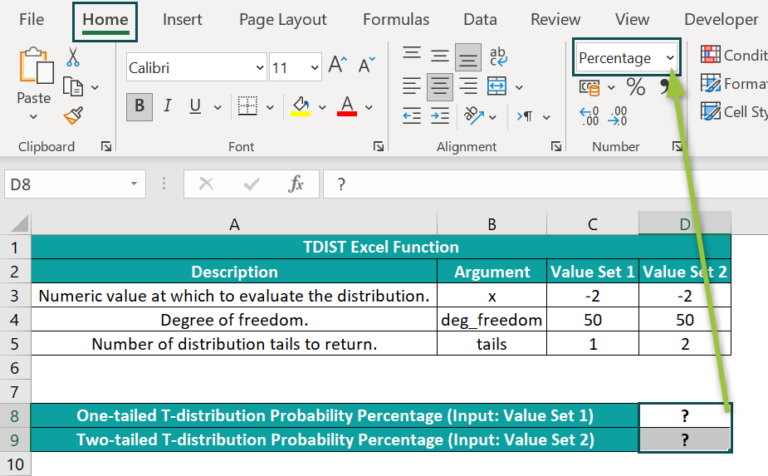Suppose we must compute and show the one- and two-tailed t-distribution probability percentages in cell range D8:D9 for the two specified value sets. And consider the target cells’ data format is Percentage.

Then, the TDIST Excel function in the target cells will fetch us the required probability values.

• Step 1: First, select the target cell D8, enter the following TDIST(), and then, press Enter.
=1-TDIST(2,50,1)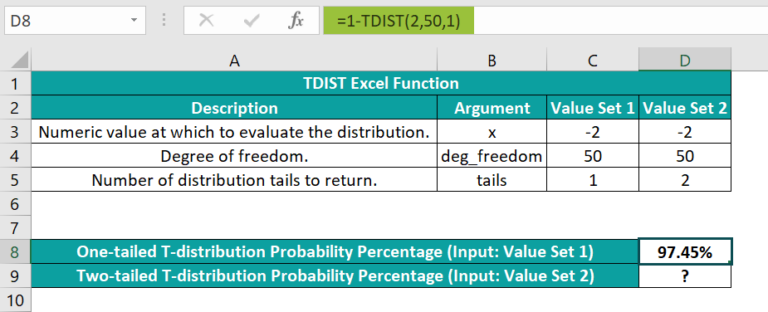• Step 2: Next, select the target cell D9, enter the following TDIST(), and press Enter.
=TDIST(2,50,2)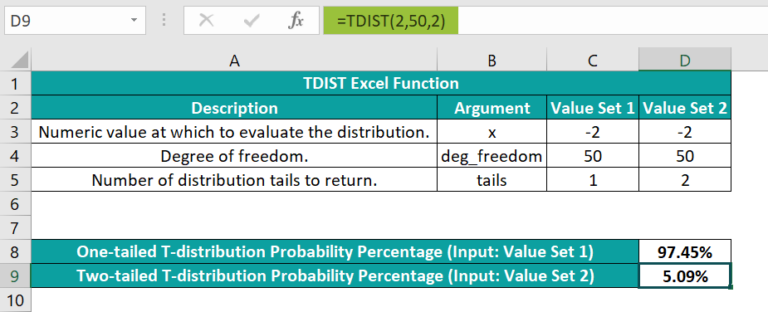In both types of t-distributions, the first argument value in the TDIST() should be positive. However, for the one-tailed t-distribution, we must subtract the TDIST() output from 1 to achieve the required P-Value. On the other hand, for two-tailed t-distribution, we can get the P-Value using the TDIST() formula we would apply when x is positive.

2. What are the methods to determine P-Value in Excel VBA?

The methods to determine P-Value in Excel VBA are as follows:

• Application.WorksheetFunction.T_Test(Arg1, Arg2, Arg3, Arg4)
• Application.WorksheetFunction.TDist(Arg1, Arg2, Arg3)

The arguments in each method have the same interpretation explained in the P-Value Formula in Excel section.

3. What is the P-Value in the Excel Regression analysis?

The P-Value in the Excel Regression analysis (for each coefficient) indicates whether the relationships in the regression model are statistically significant.
For a P-Value below the significance level, the sample data provide adequate proof to reject the null hypothesis for the whole population. It thus indicates a correlation between the regression model variables.
Otherwise, the sample data do not provide adequate evidence to infer a non-zero correlation.